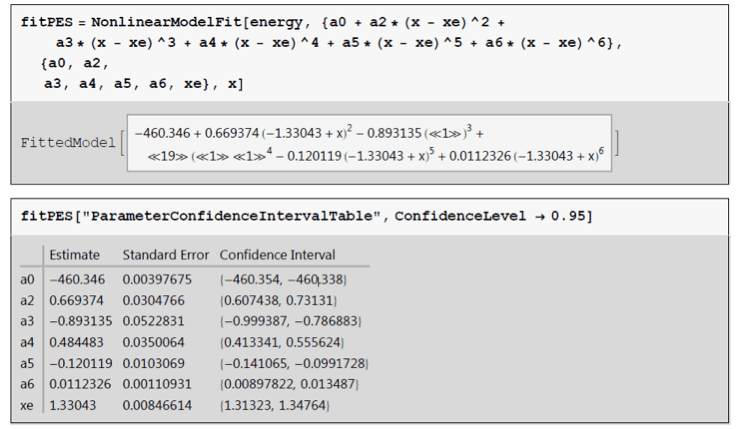# Infrared Spectrometric Rotational and Vibrational Analysis of HCl and DCl

Abstract

Infrared spectroscopy is an important analytical tool to determine the structure of molecules. Fourier transform infrared spectroscopy was used to study the vibrational and rotational motions of diatomic molecules hydrogen chloride, HCl and deuterated chloride, DCl. The isotopic effect was observed in a spectrum of both HCl and DCl with DCl at a lower wavenumber than HCl which coincided with 37Cl being observed at a lower frequency than 35Cl. The spectra of HCl and DCl were used to create separate plots of the m transition with corresponding wavenumber. Polynomial fit was determined of the plots and then used to calculate ,, De, αe, and Be by using the harmonic oscillator and rigid rotor models. These constants were then used to determine the moment of inertia, Ie, the internuclear separation, re, force constant, k, anharmonicity, vexe, and equilibrium frequency ve. The k and re were unaffected by the isotopic effect with values of 515.20 N/m and 1.31 A for HCl and 515.23 N/m and 1.30 A for DCl. Values for HCl were also determined using computational Gaussian modeling and compared to Literature. Computational and literature values had high correlation with calculated HCl constants.

Introduction

Molecules can have three modes of movement; vibration, rotation, and translation. A molecules vibrational and rotational movement is essential in the study of infrared spectroscopy, which measures the absorption of light by a molecule. Absorption of infrared light only occurs when the frequency of the wavelength is the same as the vibrational frequency of a molecule. Diatomic molecules only have one mode of vibration described by the harmonic oscillator,where E is energy, 𝑣 is the vibrational quantum number, v is frequency, and h is plank’s constant. Rotation of atoms is important in infrared study of molecules because changes in the rotational state affect the molecules vibrational fine structure. Rotation of a diatomic molecule in its simplest form is described by the rigid rotor,where J is the rotational quantum number, I is the moment of inertia, and h is plank’s constant.

Molecules undergo vibration and rotation simultaneously so Eqs. (1) and (2) are combined to describe the motion of a molecule while also considering anharmonicity and the interaction of vibration and rotation,whereis the anharmonic vibrational frequency correction, 𝐵𝑒. the rotational constant, ,𝐷𝑒 accounts for centrifugal stretching, and 𝛼𝑒 is the anharmonicity correction to rotation. Molecules are quantized so both J and 𝑣 are integers (±0, 1, 2…). At room temperture only the ground state v=0 is usually populated and Δ𝑣=+1 when excited. Rotational states have smaller energy differences than vibrational states soΔ𝐽=±1, 2, 3...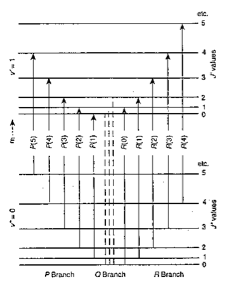Figure 1. Possible vibrational and rotational transitions.

Absorption bands vary location and intensity because of rotation-vibration interaction. A spectrum can be split into three branches P, Q, and R. The R branch represents the cumulative energy of the vibrational and rotational transitions and the P branch the difference,The Q branch is usually not observed because it represents the pure vibrational mode where rotation is Δ𝐽=0 in the excited state. Combining Eqs. (4) and (5),results in the ability to use an infrared spectrum to calculate the constants, Be,𝛼𝑒., and De of a diatomic molecule. The moment of inertia, Ie, the internuclear distance, re, force constant, k, anharmonicity, vexe, and equilibrium frequency ve can then be determined by assuming the molecule behaves as a harmonic oscillator and rigid rotor.

Vibration and rotation are contingent on the bonding molecules. Isotopic substitution changes the reduced mass, 𝜇, which effects the rotational constant, Be, and vibrational frequency,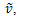The change in 𝜇 results in a different spectrum for each isotopomer. The force constant, k, and equilibrium bond length, re, are unaffected since they depend on the character of the chemical bond.

Experimental

To obtain measurements a Nicolet iS10 FTIR spectrophotometer was used in high resolution with a salt plate cell. The cell was vacuumed prior to taking experimental measurements and a baseline was obtained. The cell was then filled with HCl gas two times to ensure residual gases were removed and measurements were taken.

HCl was then reacted with D2O for 30 min to make DCl by the reaction of

𝐷2𝑂+𝐻𝐶𝑙 𝐻2𝑂+𝐷𝐻𝑂+𝐷𝐶𝑙

The D2O vial was cooled in dry ice to separate D2O/H2O from the mixture and allow HCl and DCl to fill the cell. The FTIR spectrum of the cell filled with HCl and DCl gas was taken. HCl was pumped out of the system and crystallized by a liquid nitrogen trap to prevent toxic HCl gas from entering the atmosphere.

Table 1. Reagents used

 Reagent Formula Molecular Weight (g mol-1) Density (g mL-1) CAS Number Hydrochloric Acid HCl 36.46 1.18 7647-01-0 Deuterium Chloride DCl 37.47 – 7698-05-7

Results

1.1 HCl infrared spectroscopy study

Infrared spectroscopy is a vital tool in determining quantum properties of molecules. HCl constants were determined from an IR spectrum.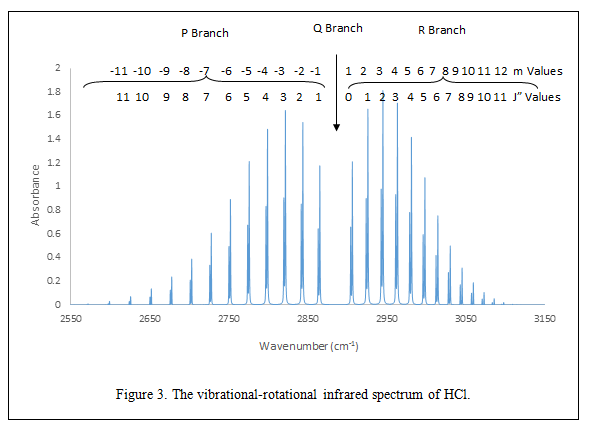Figure 3 shows the IR spectrum of HCl with rotational-vibrational effects. Absorption peak intensity increases to a maximum and then decreases moving towards. This pattern occurs due to the quantum states available and the population of atoms at that state. The distance between absorption bands,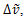in the P and R branch is expected to be 2Be and 4Be in the zero gap (Q Branch). From the spectra it is seen that moving away from the Q branch absorption bands in the P branch move apart and in the R branch closer together. The deviation from the predicted pattern occurs due to rotational-vibrational coupling and centrifugal distortion. Actual  values can be found in Table 5A under the appendix and loosely followed the expected spacing trend of 2Be and 4Be. Be is discussed in the next section and can be found in Table 2. The maxima of each absorption band was plotted against the m transition number shown in Figure 4.The second and third order polynomials were found from the data set in Figure 4 using Origin. Using the F-test it was determined that values obtained from the second order polynomial are not significantly different from values obtained from the third order polynomial. The third order polynomial was used for subsequent calculations of frequency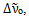, rotational constant Be, centrifugal stretching 𝐷𝑒, and the rotational anharmocity constant 𝛼𝑒. It was determined thatis 2885.4 ±0.2 cm-1 using the third order polynomial in Figure 4. The literature value foris 2885.1 cm-1 which falls outside of the calculated error, however, there is only a 0.01% difference for the values. Multiple linear regression was performed to obtain constants for HCl. Be and 𝛼𝑒.were calculated using Eq. 6 and determined to be 10.63 ± 0.09 cm-1 and 0.304 ± 0.004 cm-1, respectively. The literature value for Be of 10.54 cm-1 falls within the error of the calculated value and has a percent difference of 0.08%. 𝛼𝑒 literature value of 0.311 cm-1 is within the experimental error calculated. The De was calculated from high m transitions due to m3 dependence and found to be (7.25±0.02) x10-4 cm-1 which has an 8.2% difference with a literature value of 5.2 x10-4 cm-1. Although calculatedand De did not correlate with the literature, these values are assumed accurate since they are in the same order of magnitude with relatively small percent differences. Calculated and experiment values summarized in Table 2.

Using constants found from the third order polynomial, the anharmonic vibrational frequency correction, the equilibrium vibrational frequency ve, force constant k, internuclear distance re, and moment of inertia, Ie  were calculated. The ve andwere calculated from Eqs. 10 and 11 under the appendix, to be 2989.66 cm-1 and 52.12 cm-1, respectively. Both ve andcorrelated to literature values of 2990.95 cm-1 and 52.82 cm-1. The HCl k was found by treating the vibrational transition from the ground to first excited state as a harmonic oscillator. The k was found from Eq. 9 under the appendix to be 515.20 N/m which has a 0.07% difference with the literature value of 516.82 N/m. The re was calculated by taking HCl to resemble the rigid rotor model using Eq. 8 under the appendix to calculate experimental re at 1.31 . The re compared to the literature value of 1.27 had a 0.8% difference. The Ie was calculated to be 2.80 x 10-47 kg m2 from Eq. 7 under appendix, which correlated with the literature value of 2.64 x 10-47 kg m2 at a 1.4% difference. A summary of all HCl constants can be found in Table 2.

1.2 Computational HCl Study

Gaussian computational package was used to determine the potential energy surfaces, Figure 5, by implementing Self-Consistent Field (SCF), Second-order Møller-Plesset Perturbation Theory (MP2), and Couple Cluster with Single, Double and approximate Triple excitations (CCSD(T)).Variation among the methods is due to assumptions made in each. SCF takes the average of the electrons and ignores electron correlation. MP2 includes electron correlation effects by Rayleigh-Schrödinger perturbation theory to the second order. Among the theories used in CCSD(T) uses SCF and constructs multi-electron wavefunctions. Figure 5 to obtain the potential energy surfaces, CCSD(T) is the most accurate and was used to calculate  HCl constants. Computational constants determined by CCSD(T) for HCl correlated to both experimental and literature values with the highest percent difference at 8.2% for De between computational and experimental. The lowest percent difference was ve at 0.05%. The high correlation among the values shows the effectiveness of computational calculations using CCSD(T). A summary of all calculated computational HCl constants can be found in Table 2. Computational information under the appendix calculations.

Table 2. HCl constants with experimental calculated using the third order polynomial of Figure # compared to literature values with the percent difference and calculated computational values.

 Constant Experimental Literature7 Percent Difference [Expt. Lit.] Computational Percent Difference [Expt. Comp.](cm-1) 2885.4 ±0.2 2885.1 0.01% – – Be (cm-1) 10.63 ±0.09 10.591 0.08% 10.54 0.2% αe (cm-1) 0.304 ±0.004 0.302 0.2% 0.311 0.6% De (cm-1) (7.25 ±0.02) x10-4 5.3 x10-4 8.2% 5.2 x 10-4 8.2% ve (cm-1) 2989.66 2990.95 0.01% 2996.31 0.05% vexe (cm-1) 52.12 52.82 0.3% 52.3 1.3% k (N/m) 515.20 516.82 0.08% 518.6 0.6% re (Å) 1.31 1.27 0.8% 1.28 0.09% Ie (kg m2) 2.80 x 10-47 2.64 x 10-47 1.4% 2.65 x 10-47 0.2%

1.3 Isotopic Substitution StudyThe absorbance peak observed for H2O in Figure 6 is not relevant to this experiment and can be disregarded. Figure 6 shows the isotopic effect of 1H, 2H (D), 35Cl, and 37Cl. From the spectrum it is seen that DCl absorbed energy at a lower frequency (2000-2200 cm-1) than HCl (2600-3100 cm-1). The lower absorption frequency of DCl occurred due to a change in the reduced mass, Table 6A under the appendix, from 1.62612 x 10-27 to 1.904413 x 10-27 for HCl and DCl, respectively. It was determined that increasing the mass of an isotope resulted in absorption at a lower frequency. A shift in absorption frequency is also observed for 35Cl and 37Cl. Viewing a close-up of the spectrum in Figure 6 shows that there are two peaks present at each absorption band with 37Cl absorbing at a lower wavenumber than 35Cl. The shift of 37Cl/35Cl is small compared to the one observed for D/H which is due to a larger ratio of  for hydrogen than chlorine with values of 1.944 and 1.00, respectively.

The spectrum in Figure 6 also shows the abundance of atoms since the absorbance is directly correlated to the concentration. From the spectrum it is seen that more 35Cl than 37Cl was present which correlates to reported amounts of chlorine isotopes at 75.8% and 24.2%4. There was also more HCl than DCl present when the spectrum was taken seen by the low absorbance of DCl compared to HCl.The m transition numbers with their corresponding wavenumbers were plotted to make Figure 8. Using the F-test it was determined that values obtained from the second order polynomial are not significantly different from values obtained through the third order polynomial. Third order polynomial was used for subsequent calculations of frequency, Be, 𝐷𝑒, and 𝛼𝑒. Theis 2090.6 ±0.1 cm-1, the Be is 5.23 ±0.05 cm-1, the 𝛼𝑒 is 0.114 ±0.004 cm-1, and the De is (2.67 ±0.02) x10-4 cm-1. The lower value of Be of DCl compared to HCl represents that the absorption peaks are expected to be closer together in the DCl spectra which is observed in Figure 7 and reported in Table 5A in the appendix. The spectra of DCl also shows divergence from the 2Be and 4Be distance that was expected for.

Using constants found from the third order polynomial, the ,, the ve, the k, the re, and the Ie were calculated for DCl. Theis 26.80 cm-1 for DCl compared to 52.12 cm-1 for HCl representing that DCl needed a smaller vibrational anharmonicity correction term. The ve was found to be 2144.18 cm-1. It was expected that re would be the same for both HCl and DCl which was found to be true with re of 1.30 for DCl compared to 1.31 HCl which has a 0.2% difference. The k, which also doesn’t depend on 𝜇 only had a 0.001% difference with 515.23 and 515.20 N/m for DCl and HCl, respectively. The Ie was found to be 5.36 x10-47 which was larger than the Ie calculated for HCl. Calculated values are summarized in Table 3.

Table 3. Calculated Constants of HCl and DCl

 Constant Experimental HCl Experimental DCl µ (amu) 1.63 x10-27 3.16 x10-27(cm-1) 2885.4 ±0.2 2090.6 ±0.1 Be (cm-1) 10.63 ±0.09 5.23 ±0.05 αe (cm-1) 0.304 ±0.004 0.114 ±0.004 De (cm-1) (7.25 ±0.02) x10-4 (2.67 ±0.02) x10-4 ve (cm-1) 2989.66 2144.18 vexe (cm-1) 52.12 26.80 Ie (kg m2) 2.80 x 10-47 5.36 x10-47 re (Å) 1.31 1.30 k (N/m) 515.20 515.23

Table 4 Calculated ratios of Isotopes HCl and DCl

 Be*/Be µ/µ* (µ/µ*)1/2 ν̃e*/ν̃e 0.49 0.51 0.72 0.72

The rigid rotor prediction is proven to be accurate through comparison of Be*/Be to µ/µ* which are similar at 0.49 and 0.51. The ratio for the harmonic oscillator of (µ/µ*)1/2= ν̃e*/ν̃e was also found to be accurate with values of 0.72 for each. These ratios of HCl and DCl prove that the rigid rotor and harmonic oscillator are fairly accurate at predicting isotopic behavior.

Conclusion

The vibrational-rotational effects of HCl were explored through FTIR spectroscopy and computational methods then compared to values obtained for DCl using FTIR. Divergence from expected results was mainly due to anharmonicity and centrifugal stretching caused by vibration-rotational interaction. Experimental and computational values for HCl provided reasonable values compared to the literature. The isotopic effect was observed in the spectra of 1H, 2H, 35Cl, and 37Cl with heavier molecules absorbing at a lower frequency due to reduced mass dependence. The force constant and internuclear distance were not affected by the isotopic effect and had similar values calculated for HCl and DCl. The rigid rotor and harmonic oscillator model accurately predicted the ratios of Be and ν̃e of HCl and DCl. Studies of molecular quantum properties are important to understand how molecules will behave under varying conditions.

Works Cited

 M. Halpern and G.C. McBane, Experimental Physical Chemistry, 3rd ed., W.H. Freedman and Company, New York, 2006. Experiment 34.

 Atkins, P., J. De Paula “Physical Chemistry”, 9th ed., W. H. Freeman, New York (2010)

 Spiridoula, M.; Physical Chemistry Laboratory Molecular Constants of HCl using Computational Chemistry, Handout, Print.

 Nave, R. “Rotational Spectra.” Hyper Physics. Georgia State University, 2001. Web. 08 Apr. 2014.

 Schuder MD, Nesbitt DJ. 1994. J. Chem. Phys. 100: 7250-67

 Herzberg, G. Molecular Spectra and Molecular Structure. New York: Van Nostrand, 1950. Print

 Herzberg, G. “NIST Chemistry WebBook.” The NIST WebBook. Web. 27 October 2013.

 Cooley, J. “Rovibrational Spectroscopy.” Chemwiki. UC Davis, Web.

Appendix

Table 5A. M transitions with corresponding wavenumber for HCl and DCl used in Figures 3 and 7 and calculated Δν.

 HCl DCl m v (cm-1) Δν (cm-1) m v (cm-1) Δν (cm-1) -11 2625 27 -9 1986 12 -10 2652 25 -8 1998 12 -9 2677 25 -7 2010 13 -8 2702 25 -6 2023 11 -7 2727 25 -5 2034 12 -6 2752 23 -4 2046 11 -5 2775 23 -3 2057 12 -4 2798 23 -2 2069 11 -3 2821 22 -1 2080 (Zero Gap) 21 -2 2843 20 1 2101 11 -1 2863 (Zero Gap) 43 2 2112 10 1 2906 20 3 2122 9 2 2926 18 4 2131 10 3 2944 19 5 2141 9 4 2963 17 6 2150 9 5 2980 18 7 2159 9 6 2998 16 8 2168 9 7 3014 16 9 2177 9 8 3030 15 10 2186 9 3045 14 10 3059 11 11 3070 15 12 3085

Table 6A. Reduced mass and reduced mass ratios for isotopic molecules

 Molecule Reduced Mass, 𝜇 (kg) Isotopes Reduced Mass Ratio 1H35Cl 1.62612 x 10-27 1H35Cl / 1H37Cl 1.002 1H37Cl 1.62859 x 10-27 2H35Cl 1.904413 x 10-27 1H35Cl / 2H35Cl 1.944 2H37Cl 1.910033 x 10-27

CalculationsComputational calculations for HCl